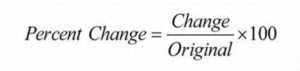## MS Math – Nov 29-Dec 3We hope you had a wonderful Thanksgiving!

6th Grade Math – Mrs. Evans

This week we will continue the study of ratios, rates and unit rates in Chapter 4. Rates and unit rates are very useful mathematical concepts that we use in day to day life, such as when we buy things (\$/packages) or maintain a safe speed in the car (miles/hour).  Take the opportunity to discuss as many of these situations that your family encounters this week!  Here is a video to help your student understand Ratios, Rates and Unit Rates:

Math Grade Math – Mrs. Evans and Mrs. VonFeldt

This week, we will do what we had planned to do last week – start Module 5!    We will learn about finding the percent change in different amounts.  I include this from last week because it did not get covered last week.We will be determining the markup and markdown on quantities using percentages.  Finding markup and markdown requires thinking logically.  Here is a video about percent change to introduce you to this useful concept.

We will use this concept in solving real world problems.  As a family you can call attention to all the places that we use percentages in day to day life; when we shop, eat out, leave a tip, pay taxes, make interest on our savings or pay interest on loans.  It is a great time to talk about these situations with your student!

8th Grade Math – Mrs. Ernest

The students are learning how to apply exponent rules to Scientific Notation this week! We will begin by learning the Scientific Notation format, then we will practice converting large and small numbers into Scientific Notation. Next, we will work backwards- turning a number written in the notation back to a large or small number. Students will learn how to multiply and divide Sci Notation numbers, and if we have time, we will begin to apply all of our learning about exponents to a new concept: Square Roots!

Algebra – Mrs. VonFeldt

This week, we will continue to practice writing and graphing linear equations. Students will learn how to express a line in point-slope form. This form is particularly helpful when you are given a slope and a random point on the line. Then, they will learn how to rewrite that equation into slope-intercept form or standard form. Students should be able to go back and forth between these three forms by rewriting the equation.

## MS Math – Nov 15-19, 20216th Grade Math – Mrs. Evans

This week we will finish with graphing rational numbers in Chapter 3 with a test on Wednesday.  We will begin to investigate ratios, rates and unit rates as we work into Chapter 4.  Here is a video about ratios and rates:

Math Grade Math – Mrs. Evans and Mrs. VonFeldt

This week, we will finish our study of proportional relationships with a heavy quiz on  Thursday.  We have learned a lot in this module and will be able to apply it throughout the rest of the year.  In Module 5 we will plunge into studying about percents and how to determine a percent change.  The percent change is defined as follows:We will be determining the markup and markdown on quantities using percents.  Here is a video about percent change to introduce you to this useful concept.

8th Grade Math – Mrs. Ernest

Algebra – Mrs. VonFeldt

There will be a Chapter 3 test on Thursday, November 18. It will cover graphing linear equations. In Chapter 4 we will look at ways to find the equation of a line when you have different pieces of information. How can you find an equation when you have two points? A point and a slope? A y-intercept and a point? Students are getting very familiar with the different representations of linear situations. They can now graph directly from an equation, create a table from an equation or graph, and find an equation directly from a graph.

## MS Math – Nov 8-12, 20216th Grade Math – Mrs. Evans

We will only have 3 days of math this week as Thursday is Veterans day and Friday the 6th graders will be doing a service project.

This week will explore graphing on the coordinate system.  We will graph ordered pairs of rational numbers and analyze some interesting things about them, like quadrants, line symmetry and distance between points.  Here is a picture of the coordinate plane:Math Grade Math – Mrs. Evans and Mrs. VonFeldt

This week, as we work in Module 4, we will continue to learn about how to write proportional equations, how to graph them, what they look like when on a graph and how to analyze a graph for information about the proportional relationship.  Here is a video from Khan Academy about writing proportional equations:

And here is another video from Khan Academy about graphing proportional equations:

8th Grade Math – Mrs. Ernest

Students have been learning about linear equations (y=mx+b). This unit we will transition to thinking about more complex equations, like quadratics! Students will be introduced to the exponent rules and learn more about algebra with exponents as we move forward.

Algebra – Mrs. VonFeldt

We are continuing to explore how linear functions can be written and graphed. This week we will look at proportional relationships. Proportional relationships are just linear functions that have a common ratio called the constant of proportionality, k. The graphs go through the origin. See the Khan Academy videos above in 7th grade for review.

This week we will also learn function notation. Function notation is a fancy algebraic way of writing “y”.The following Math Antics video is an excellent summary of what we have learned so far about linear functions:

© 2023 Assumption Catholic School | 2116 Cornwall Ave, Bellingham, WA 98225
Phone: 360.733.6133 | Fax: 360.647.4372
Email: theoffice@school.assumption.org
Dashboard | Web design and development by Olywebdev.com